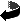# Regularity for eigenfunctions of Schrödinger operators by Bernd Ammann, Catarina Carvalho and Victor Nistor

Regularity for eigenfunctions of Schrödinger operators (.dvi, .ps,.ps.gz or .pdf)
Lett. Math. Phys. 101, 49-84 (2012)
DOI 10.1007/s11005-012-0551-z

### Abstract

We prove a regularity result for the eigenfunctions of a single nucleus Schrödinger operator in weighted Sobolev (or Babuska--Kondratiev) spaces. More precisely, if Kam is the weighted Sobolev space obtained by desingularization of the set of singular points of the potential
V(x)= ∑1 ≤ j ≤ N \frac{bj}{|xj|} + ∑1 ≤ i < j ≤ N \frac{cij}{|xi-xj|},
x ∈ R3N, bj, cij ∈ R,
and (-Δ + V) u = λ u, u ∈ L2(R3N}), then u ∈ Kam for all m ∈ Z+ and all a ≤ 0.
Our result extends to the case when bj and cij suitable bounded functions.

### Typos in the published version

• In Definition 2.3: In the phrase "(which is equivalent to saying that $\bar x$ is in the interior of $X\cap H_1\cap \ldots \cap H_s$)" one should replace "$X\cap H_1\cap \ldots \cap H_s$" by "$H_1\cap \ldots \cap H_s$".
• In Subsection 3.5 the typesetter of the journal inserted some typos. At several places tilde symbols were inserted in the text, although they should have been inserted in the source code for better line breaks. One should just remove these symbols. This only affects the published version. In particular, one should twice remove a tilde before the submanifold X. Similar problems have been inserted before the open subset U and also in other subsections before other symbols.

### A comment on Prop. 2.5

In the article we mostly restricted to blow-up along manifolds of positive codimension. Nevertheless most statement are still true if we allow blow-ups along manifolds of codimension 0. If X is of codimension 0 in M, then [M:X]=M\X. Thus one immediatly sees that Proposition 3.2 is still true in the case that X has the same dimension as Y. However, as this subtle case is not needed we avoided this discussion in the article.Zurück zur Homepage

The Paper was written on 24.9.2010
Last update 5.4.2013, tiny addition on web page 5.8.2021